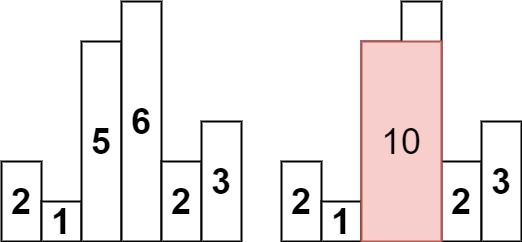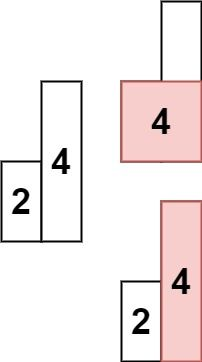# Question

Formatted question description: https://leetcode.ca/all/84.html

Given an array of integers heights representing the histogram's bar height where the width of each bar is 1, return the area of the largest rectangle in the histogram.

Example 1:Input: heights = [2,1,5,6,2,3]
Output: 10
Explanation: The above is a histogram where width of each bar is 1.
The largest rectangle is shown in the red area, which has an area = 10 units.


Example 2:Input: heights = [2,4]
Output: 4


Constraints:

• 1 <= heights.length <= 105
• 0 <= heights[i] <= 104

# Algorithm

### keyword: “Eliminate the shadow”, that is, the lowest bar is the length of a side

Intuitively, the area of the two bars depends on the shortest and lowest bar among them.

My own doubt is if it is [1,2,3,4,1,2,3,4].

How to prove that the first 1 and last 4 restricted rectangles will definitely not be the largest? Because the stack only maintains the current one, note that there is only one, an increasing sequence.

Simplified to [1,2,1,2], then the maximum area is the area 4 formed by the first 1 and the last 2

• So the key is that the push and pop conditions of the stack must ensure that the lowest bar is always in the stack.

Extend to eg. [3,1,2,1,2], the largest area also comes from the area formed by the first and last bar at 5.

Because the lowest one will always be in the stack, and the stack will eventually pop to empty, The last pop is the smallest bar. At this time, the shortest bar is multiplied by the length from the first to the last.

The whole question will be clear if you understand this: int currentWidth = sk.isEmpty()? i: (i-1-sk.peek());

sk.peek() is definitely the essence of the quintessence, to find the width of the largest rectangle, I wrote i-currentIndex at the beginning, but I ignored the situation when counting 4 in 2, 8, 8, 4, 6, 8. There are two 88 in front of 4, that is, the width should be from the first 8 to the last 8. At this time, to find this width, you need sk.peek() to find the previous small index

There is another pit, which is the application of dummy end, which has the same effect as dummy head, allowing the for loop to start and run normally.

Arrays.copyOfRange(origin, start, end), if end exceeds the length of the origin array, 0 will be added automatically

The best test case:

• 2,1,2
• 2,0,2
• 2,0,2,1,2

# Code

• import java.util.Arrays;
import java.util.Stack;

public class Largest_Rectangle_in_Histogram {

public int largestRectangleArea(int[] rowarray) {

// stack is to push array index of each element
Stack<Integer> sk = new Stack<Integer>();

// length+1, in the end add a dummy element as 0
int[] row = new int[rowarray.length + 1];
// @note: Arrays.copyOf()
row = Arrays.copyOf(rowarray, rowarray.length + 1);

int max = 0;

// for (int i = 0; i < row.length; i++) {
int i = 0;
while (i < row.length) {

// @note: careful about duplicate numbers being pushed
//          "<=" and "<" both AC, since same height bar will be popped and checked anyway, just a matter of when popped
// if (sk.isEmpty() || row[sk.peek()] <= row[i]) { @note: this is AC also
if (sk.isEmpty() || row[sk.peek()] < row[i]) {
sk.push(i);
i++;
} else { // value of index-i is smaller than current stack top

// @note:  to get lowest bar, since other bars are all larger than it
int currentLowestBarIndex = sk.pop();
int edge1 = row[currentLowestBarIndex];
// @note: empty meaning just popped lowest bar
// @note: sk.peek() is
int edge2 = sk.isEmpty() ? i : (i - 1 - sk.peek()); // i-1-peek is ok, since it's monotonic increasing stack

// calculate area
max = Math.max(max, edge1 * edge2);
}
}

return max;
}

}

############

class Solution {
public int largestRectangleArea(int[] heights) {
int res = 0, n = heights.length;
Deque<Integer> stk = new ArrayDeque<>();
int[] left = new int[n];
int[] right = new int[n];
Arrays.fill(right, n);
for (int i = 0; i < n; ++i) {
while (!stk.isEmpty() && heights[stk.peek()] >= heights[i]) {
right[stk.pop()] = i;
}
left[i] = stk.isEmpty() ? -1 : stk.peek();
stk.push(i);
}
for (int i = 0; i < n; ++i) {
res = Math.max(res, heights[i] * (right[i] - left[i] - 1));
}
return res;
}
}

• // OJ: https://leetcode.com/problems/largest-rectangle-in-histogram/
// Time: O(N)
// Space: O(N)
// Ref: https://leetcode.com/problems/largest-rectangle-in-histogram/discuss/28902/5ms-O(n)-Java-solution-explained-(beats-96)
class Solution {
public:
int largestRectangleArea(vector<int>& A) {
int N = A.size(), ans = 0;
vector<int> prevSmaller(N, -1), nextSmaller(N, N);
for (int i = N - 2; i >= 0; --i) {
int j = i + 1;
while (j < N && A[j] >= A[i]) j = nextSmaller[j];
nextSmaller[i] = j;
}
for (int i = 1; i < N; ++i) {
int j = i - 1;
while (j >= 0 && A[j] >= A[i]) j = prevSmaller[j];
prevSmaller[i] = j;
}
for (int i = 0; i < N; ++i) ans = max(ans, (nextSmaller[i] - prevSmaller[i] - 1) * A[i]);
return ans;
}
};

• '''
枚举每根柱子的高度 h 作为矩形的高度，向左右两边找第一个高度 小于(<) h 的下标 left_i, right_i

那么此时矩形面积为 h * (right_i - left_i - 1)，求最大值即可。
'''
class Solution:
def largestRectangleArea(self, heights: List[int]) -> int:
n = len(heights)
stk = []
left = [-1] * n
right = [n] * n
for i, h in enumerate(heights):
while stk and heights[stk[-1]] >= h:
right[stk[-1]] = i
stk.pop()
if stk:
left[i] = stk[-1] # same as below: stk[-1] in 'i - stack[-1] - 1'
stk.append(i)
return max(h * (right[i] - left[i] - 1) for i, h in enumerate(heights))

############

class Solution:
def largestRectangleArea(self, heights: List[int]) -> int:
if not heights:
return 0
heights.append(-1) # make for loop running
stack = []
ans = 0
for i in range(0, len(heights)):
while stack and heights[i] < heights[stack[-1]]:
currentLowestBarIndex = stack.pop()
h = heights[currentLowestBarIndex]
# stack[-1] is after pop(). why not i-currentLowestBarIndex?
# because not working for tie case in the stack,
# eg. for input=[4,2,0,3,2,5], expected=6 but output=5
# 向左右两边找第一个高度 小于(<) h 的下标 left_i, right_i
#   如果是左右两边都是 大于 h 的下标，那么宽度是 right-left+1
#   但是这里是 小于 h的下标，right-left+1 情况 向左向右 都拓展一个index，多了两个，+1 -2 得 -1
w = i - stack[-1] - 1 if stack else i
ans = max(ans, h * w)
stack.append(i)

heights.pop() # restore, pop -1
return ans

• func largestRectangleArea(heights []int) int {
res, n := 0, len(heights)
var stk []int
left, right := make([]int, n), make([]int, n)
for i := range right {
right[i] = n
}
for i, h := range heights {
for len(stk) > 0 && heights[stk[len(stk)-1]] >= h {
right[stk[len(stk)-1]] = i
stk = stk[:len(stk)-1]
}
if len(stk) > 0 {
left[i] = stk[len(stk)-1]
} else {
left[i] = -1
}
stk = append(stk, i)
}
for i, h := range heights {
res = max(res, h*(right[i]-left[i]-1))
}
return res
}

func max(a, b int) int {
if a > b {
return a
}
return b
}

• using System;
using System.Collections.Generic;
using System.Linq;

public class Solution {
public int LargestRectangleArea(int[] height) {
var stack = new Stack<int>();
var result = 0;
var i = 0;
while (i < height.Length || stack.Any())
{
if (!stack.Any() || (i < height.Length && height[stack.Peek()] < height[i]))
{
stack.Push(i);
++i;
}
else
{
var previousIndex = stack.Pop();
var area = height[previousIndex] * (stack.Any() ? (i - stack.Peek() - 1) : i);
result = Math.Max(result, area);
}
}

return result;
}
}